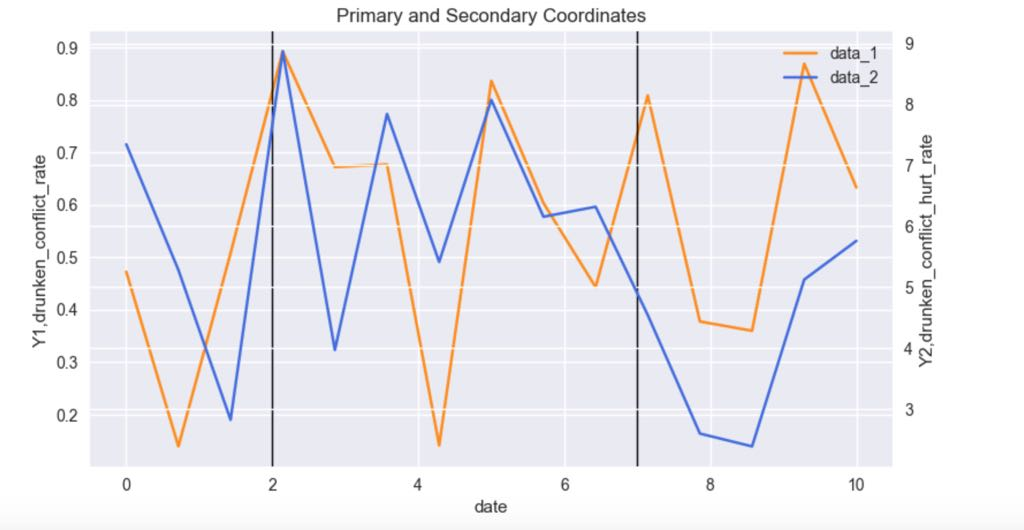# Python绘制主次坐标图2018年7月11日17:51:59

2 1695字阅读5分39秒

## 主次坐标图绘制

1. import numpy as np
2. import pandas as pd
3. from matplotlib import pyplot as plt
4. from matplotlib.lines import Line2D
5. import seaborn as sns
6. %matplotlib inline
7. # ----------
8. # 生成样例数据
9. # ----------
10. x = np.linspace(0,10,15)
11. y_data1 = np.random.rand(15)
12. y_data2 = 5 * y_data1 + 5 * np.random.rand(15)
13. # -------
14. # 进行绘图
15. # -------
16. sns.set()
17. # 画出双坐标轴图像
18. fig, ax1 = plt.subplots(figsize=(9, 5),dpi=100)
19. ax2 = ax1.twinx()
20. # 这里获取图为了后面添加图例
21. l1, = ax1.plot(x, y_data1, 'darkorange')
22. l2, = ax2.plot(x, y_data2, 'royalblue')
23. # 设置x,y轴文字说明
24. ax1.set_xlabel('date')
25. ax1.set_ylabel('Y1,drunken_conflict_rate')
26. ax2.set_ylabel('Y2,drunken_conflict_hurt_rate')
27. # 设置图例
28. plt.legend(handles = [l1, l2,], labels = ['data_1', 'data_2'], loc='best')
29. # 设置标题
30. ax1.set_title('Primary and Secondary Coordinates')
31. # -------
32. # 绘制直线
33. # -------
34. # 两条line的数据
35. line1 = [(2, 0), (2, 1)]
36. line2 = [(7, 0), (7, 1)]
37. (line1_xs, line1_ys) = zip(*line1)
38. (line2_xs, line2_ys) = zip(*line2)
39. # 创建两条线，并添加
42. # 显示图像
43. plt.show()### 主次坐标轴绘制图例

1. fig.legend(fontsize=17, bbox_to_anchor=(0.83, 0.87))

## 结语• 微信公众号
• 关注微信公众号
•• QQ群
• 我们的QQ群号
•• 本文由 发表于 2018年7月11日17:51:59
• 转载请务必保留本文链接：https://mathpretty.com/9441.html